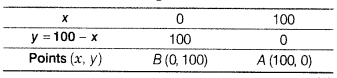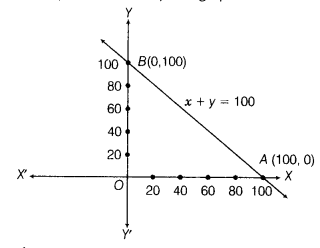# Yamini and Fatima, two students of Class IX of a school, together contributed ₹ 100

Yamini and Fatima, two students of Class IX of a school, together contributed ₹ 100 towards the Prime Minister’s Relief Fund to help the earthquake victims. Write a linear equation which satisfies this data. (You may take their contributions as ₹ x and ₹ y). Draw the graph of the same.

Let the contributions of Yamini and Fatima towards the Prime Minister’s Relief Fund to help the earthquake victims be ₹ x and ₹ y, respectively.
Then, by given condition, we have x + y = 100 … .(i)
Now, we have the following tableNow, plot the points B(0,100) and A(100, 0)on the graph paper and join all these points to form a line AB which represents the required graph,